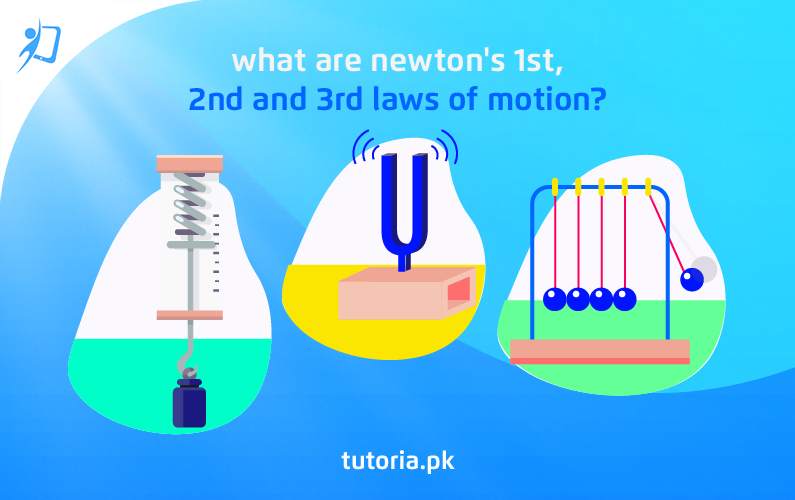# What are Newton’s 1st, 2nd and 3rd Laws of Motion?

September 10, 2020 | intermediate students | 0 commentsAll pre-medical and pre-engineering intermediate students alike can relate to the complex subject that is physics. For most students, physics requires a huge amount of self-studying and past papers practice in order to understand. For other students, even that isn’t enough to gain command over the subject.

In physics, some topics are fundamental to the subject. Therefore, students must have clear concepts of these fundamental topics, in order to perform well on their final Lahore Board physics exam. One such topic is Newton’s Three Laws of Motion.

### How to Learn Newton’s Three Laws of Motion

For the best exam preparation, intermediate students should always turn to tutoria.pk. It provides the highest quality exam study resources for students of all classes, subjects, and educational boards.

Therefore, at tutoria.pk, students can gain access to top-quality physics book notes, previous five-year physics Lahore Board solved past papers, lecture videos, and more. This allows students to develop a strong understanding of physics concepts, such as Newton’s Three Laws of Motion. In addition, solving the Lahore Board solved past papers will effectively prepare students for the final exams.

To help you get started, here is an overview of Newton’s 1st, 2nd, and 3rd laws of motion:

##### Newton’s 1st Law of Motion

Newton’s 1st Law of Motion states that:

“An object at rest remains at rest, or if in motion, remains in motion at a constant velocity unless acted on by a net external force.”

This is commonly also referred to as the Law of Inertia. In simple words, it means that if an object is at rest, it will remain at rest, until it is acted upon by a force. Similarly, if the object is moving at a constant speed in a straight line, it will continue to keep moving in a straight line at constant speed unless it is acted upon by a force.

##### Newton’s 2nd Law of Motion

Newton’s 2nd Law of Motion states that:

“The acceleration of an object as produced by a net force is directly proportional to the magnitude of the net force, in the same direction as the net force, and inversely proportional to the mass of the object.”

This is arguably one of the most important laws in your physics textbook. It is used in almost every physics chapter and frequently appears in the past papers.  It explains exactly how much an object will accelerate for a given net force.

##### Newton’s 3rd Law of Motion

Newton’s 3rd Law of Motion states that:

“If an object A exerts a force on object B, then object B must exert a force of equal magnitude and opposite direction back on object A.”

This is another integral concept in the subject of physics. This law represents a certain symmetry in nature: forces always occur in pairs, and one body cannot exert a force on another without experiencing a force itself.

For a more detailed explanation of Newton’s Three Laws of Motion, be sure to subscribe to tutoria.pk today and learn from our expert teacher’s online lectures, physics book notes, and five-year solved past papers!